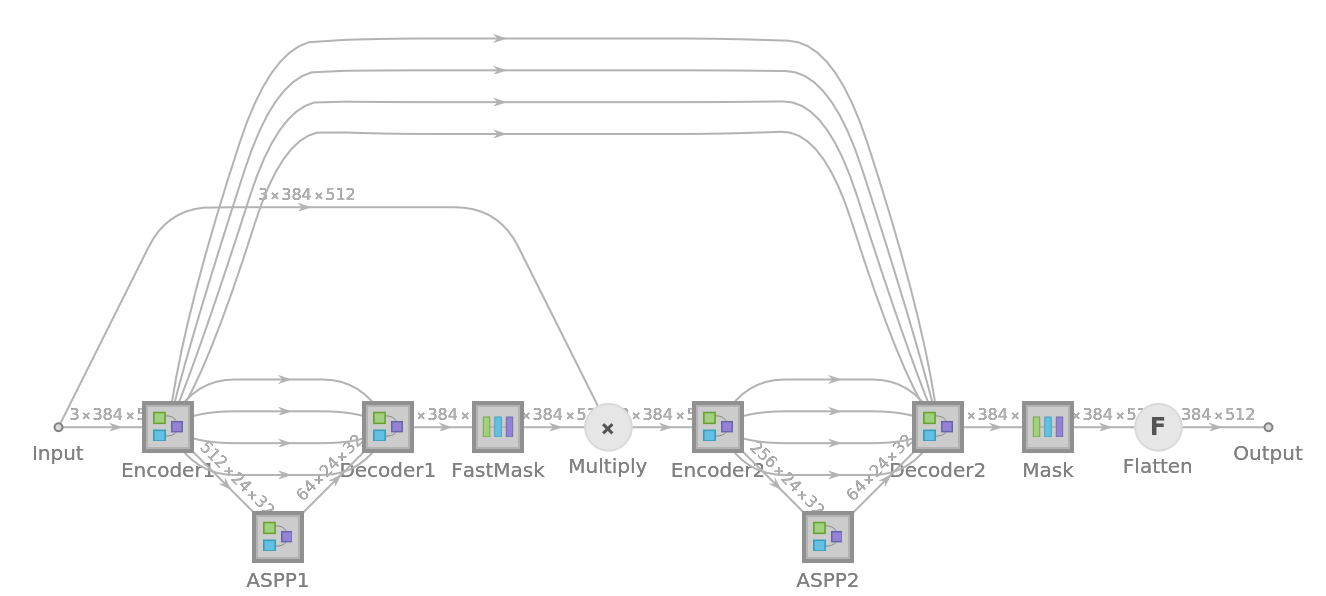# DoubleU-Net Trained onMedical Image Segmentation Datasets

Segment medical images performing a task-dependent binary classification

The authors propose a novel architecture called DoubleU-Net, combining two U-Net blocks stacked on top of each other. The first U-Net works as an encoder, using a VGG-19 pre-tained on the ImageNet dataset, while the second U-Net is added to capture more semantic information. An atrous spatial pyramid pooling (ASPP) block is added to capture contextual information within the network. The architecture was trained over different medical image segmentation datasets covering areas such as colonoscopy, dermoscopy and microscopy. The result outperforms U-Net for all the tasks.

## Training Set Information

• Three datasets where used to train and evaluate the effectiveness of the models: the CVC-ClinicDB dataset for polyp segmentations, the lesion boundary segmentation dataset of the ISIC 2018 challenge for skin lesions and the data from the 2018 Data Science Bowl challenge for nuclei segmentation.

## Model Information

•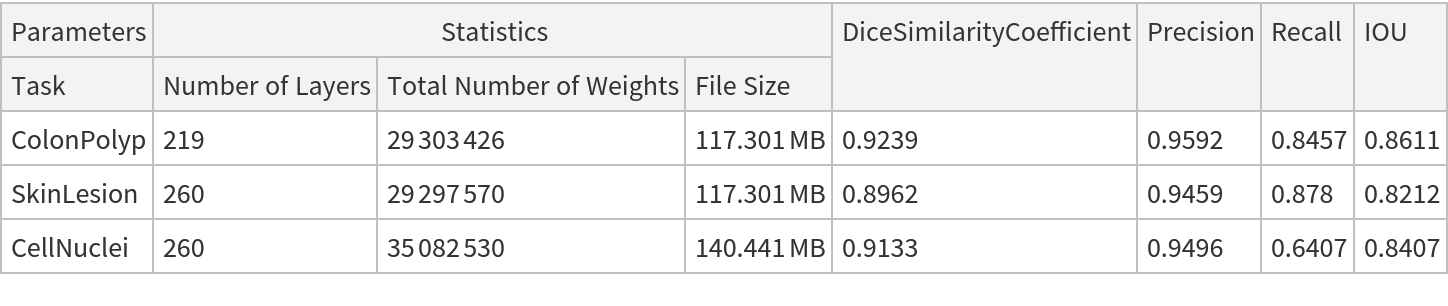## Examples

### Resource retrieval

Get the pre-trained net:

 In:=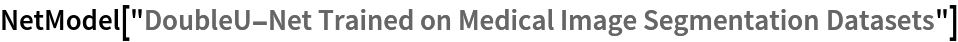Out=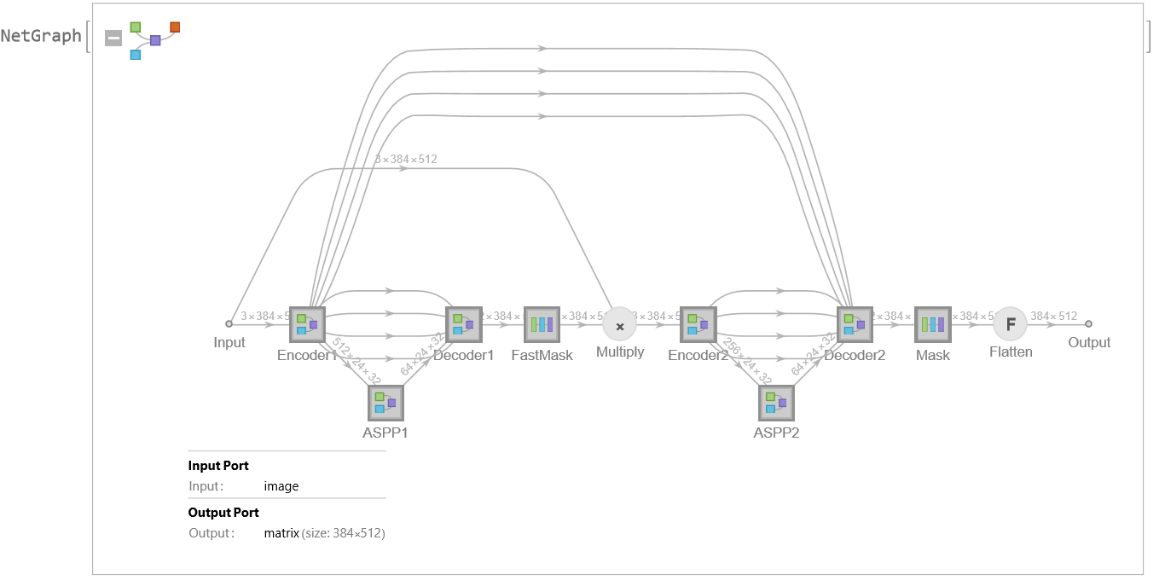### NetModel parameters

This model consists of a family of individual nets, each identified by a specific parameter combination. Inspect the available parameters:

 In:=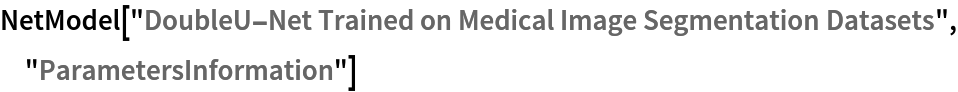Out=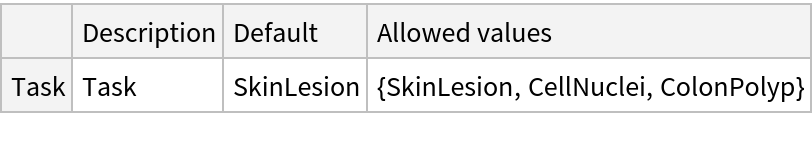Pick a non-default net by specifying the parameters:

 In:=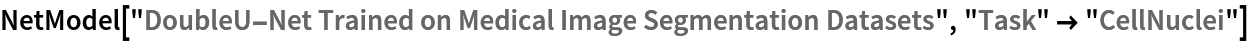Out=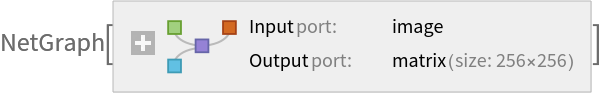Pick a non-default uninitialized net:

 In:=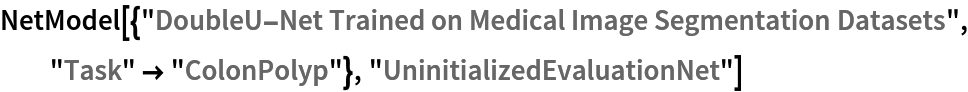Out=### Evaluation function

Define an evaluation function to select the evaluation mode and postprocess the net output:

 In:=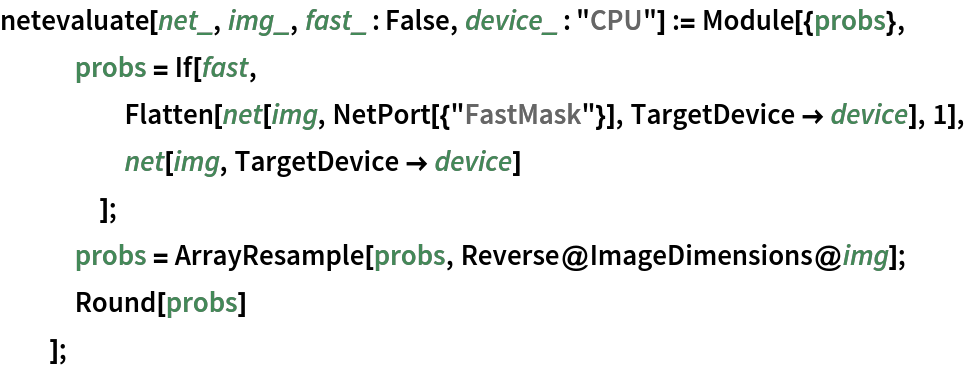### Basic usage

Obtain the segmentation mask of a skin lesion:

 In:=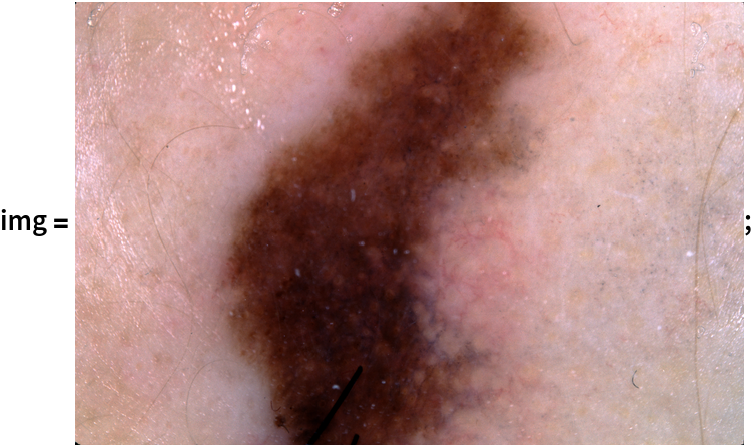In:=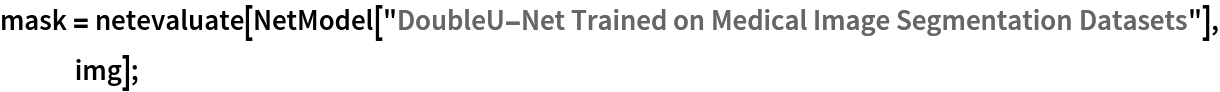In:=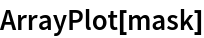Out=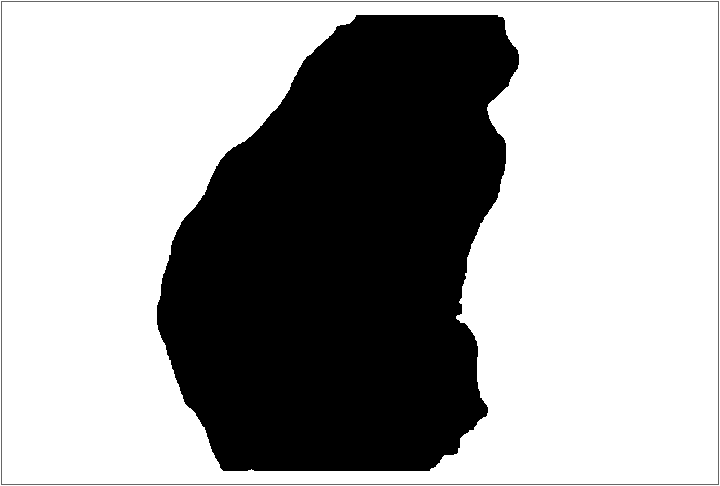The mask is a matrix of 0 and 1 whose size matches the dimensions of the input image:

 In:=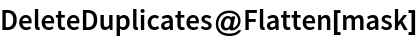Out=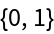In:=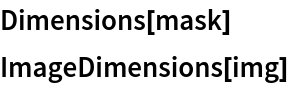Out=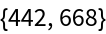Overlay the mask on the input image:

 In:=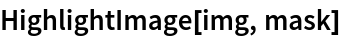Out=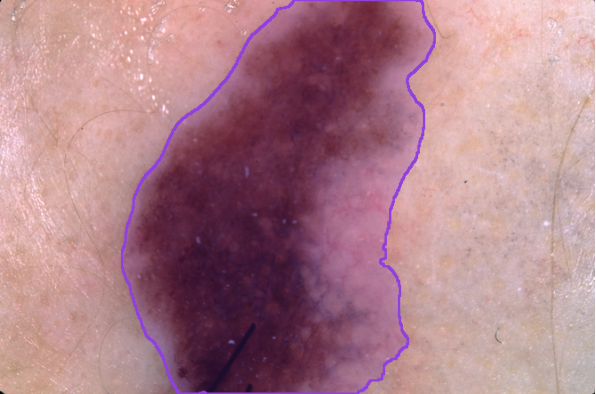The nets compute a lower-quality segmentation mask as an intermediate step. It is possible to stop the computation at such intermediate step to obtain a segmentation mask faster. This can be enabled by setting the third argument of the evaluation function to True:

 In:=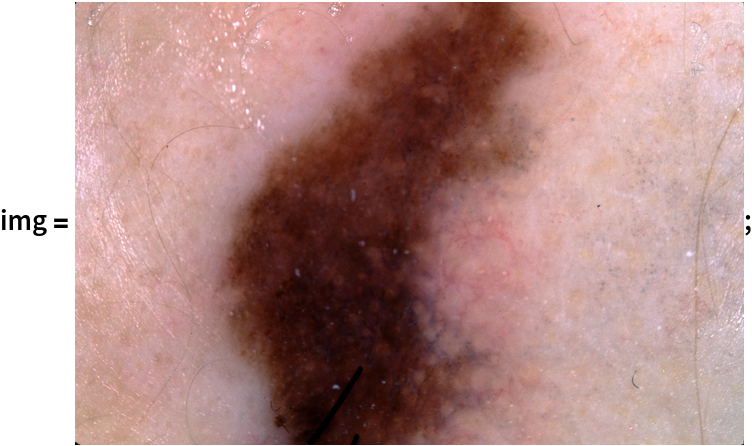In:=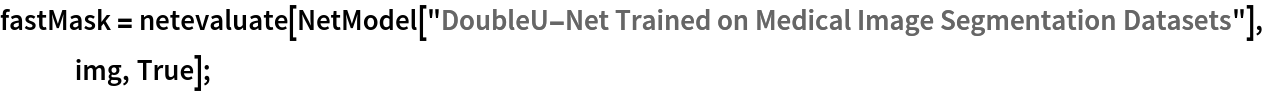In:=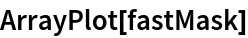Out=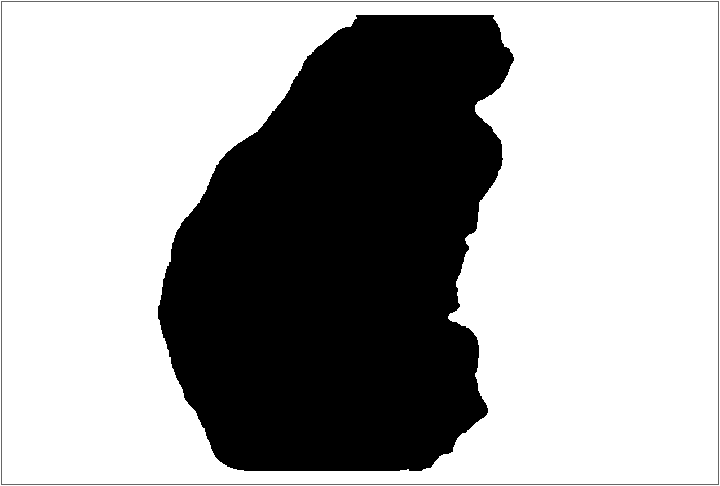Overlay the mask on the input image:

 In:=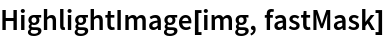Out=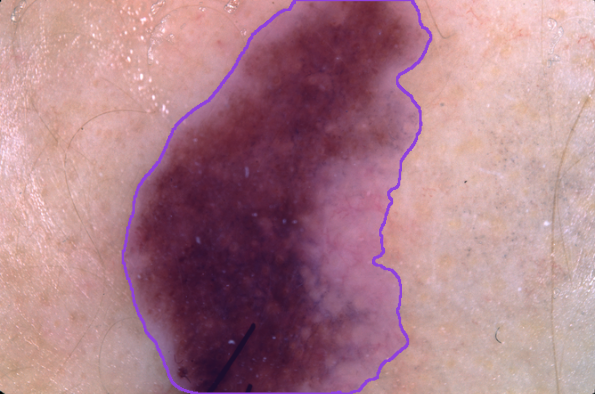Compare the evaluation timings of the fast and slow masks:

 In:=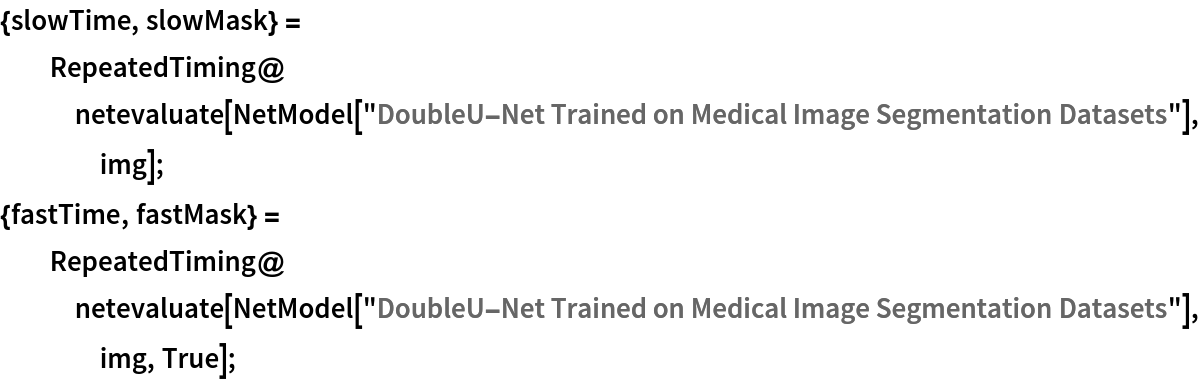In:=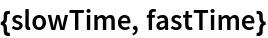Out=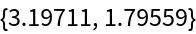Compare the quality of the two segmentation masks. The fast mask is in blue and the slow mask is in red:

 In:=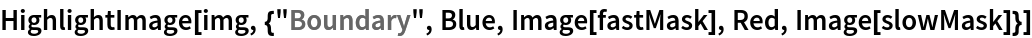Out=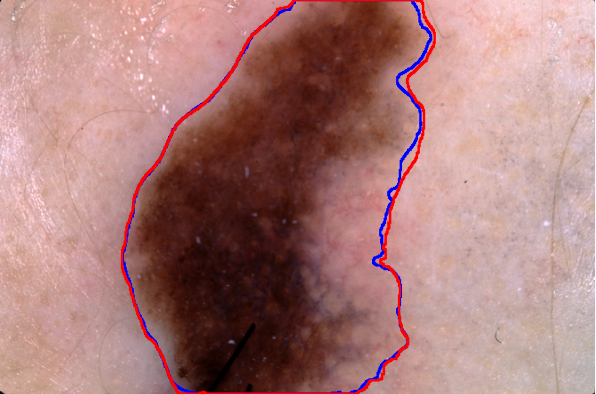### Net information

Obtain the total number of parameters:

 In:=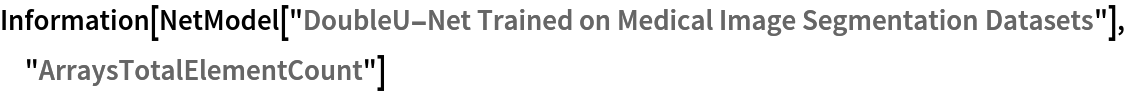Out=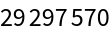Obtain the layer type counts:

 In:=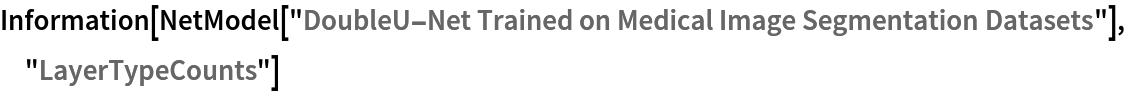Out=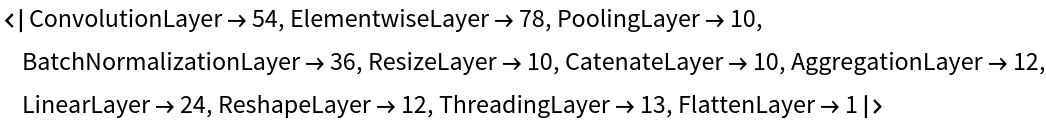Display the summary graphic:

 In:=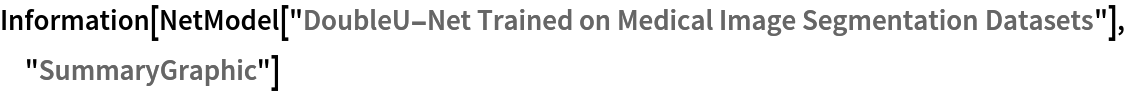Out=